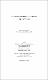## On non-vanishing of certain L-functions2003
##### Authors
Shahabi, Shahab
University of Lethbridge. Faculty of Arts and Science
##### Publisher
Lethbridge, Alta. : University of Lethbridge, Faculty of Arts and Science, 2003
##### Abstract
This thesis presents the following: (i) A detailed exposition of Rankin's classical work on the convulsion of two modular L-functions is given; (ii) Let S be the calss Dirichlet series with Euler product on Re(s) > 1 that can be continued analytically to Re(s) = 1 with a possible pole at s = 1. For F,G E S, let F X G be the Euler product convolution of F and G. Assuming the existence of analytic continuation for certain Dirichlet series and some other conditions, it is proved that F x G is non-vanishing on the line Re(s) = 1; (iii) Let Fn be the set of newforms of weight 2 and level N. For f E Fn, let L(sym2f,s) be the associated symmetric square L-function. Let s0=0o + ito with 1 - 1/46 < 0o <1. It is proved that Cs0,EN1-E<#{f E Fn; L (sym2 f, so)=0} for prime N large enough. Here E>0 and Cso,E is a constant depending only on So and E.
##### Description
vii, 78 leaves ; 29 cm.
##### Keywords
L-functions , Number theory , Dissertations, Academic